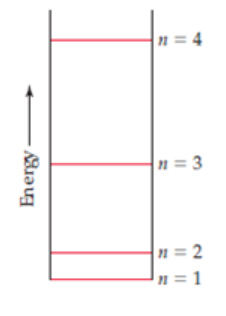# Problem: A certain quantum mechanical system has the energy levels shown in the accompanying diagram. The energy levels are indexed by a single quantum number n that is an integer, (a) As drawn, which quantum numbers are involved in the transition that requires the most energy?(b) Which quantum numbers are involved in the transition that requires the least energy?(c) Based on the drawing, put the following in order of increasing wavelength of the light absorbed or emitted during the transition: (i) n = 1 to n = 2(ii) n = 3 to n = 2(iii) n = 2 to n = 4(iv) n = 3 to n = 1

###### FREE Expert Solution

↑ E → ↓ λ

Based on the diagram, the order of increasing energy of the given transitions are as follow

84% (204 ratings)###### Problem Details

A certain quantum mechanical system has the energy levels shown in the accompanying diagram. The energy levels are indexed by a single quantum number n that is an integer,

(a) As drawn, which quantum numbers are involved in the transition that requires the most energy?

(b) Which quantum numbers are involved in the transition that requires the least energy?

(c) Based on the drawing, put the following in order of increasing wavelength of the light absorbed or emitted during the transition:

(i) n = 1 to n = 2

(ii) n = 3 to n = 2

(iii) n = 2 to n = 4

(iv) n = 3 to n = 1Frequently Asked Questions

What scientific concept do you need to know in order to solve this problem?

Our tutors have indicated that to solve this problem you will need to apply the Bohr Model concept. You can view video lessons to learn Bohr Model. Or if you need more Bohr Model practice, you can also practice Bohr Model practice problems.

What professor is this problem relevant for?

Based on our data, we think this problem is relevant for Professor Czernuszewicz's class at UH.